## The most complicated Equation you can think of

Things that are not directly connected with Factorio.
Mrmeguyme-
Burner Inserter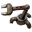Posts: 7
Joined: Thu Feb 23, 2017 9:45 pm
Contact:

### The most complicated Equation you can think of

Try and come up with the most complicated equation you can think of.

keyboardhack
Filter Inserter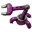Posts: 473
Joined: Sat Aug 23, 2014 11:43 pm
Contact:

### Re: The most complicated Equation you can think of

I cheated. My computer came up with this one. You may get an error if you try run the function depending on the precision of the program running it.

f(x) = 1+(cos(ln(x/exp((x+x^arccos(sqrt(1/(arcsin(ln(tan(sqrt(ln(exp(2*x/(Pi-2*arccos(cos(arcsin(x)^2/(1+arcsin(x)^2)))))-x)/ln(10)))+1)/ln(10))+tan(sin(x^(1/sqrt(1+ln((1/2)*Pi-1)^2/ln(10)^2)-1)))-tan(ln(ln(-arctan(x*(arcsin(x*tan(exp(exp(1))))-1))))*sqrt(exp(x/sqrt(sin(exp(1)*x)))+arctan(arctan((x^arctan((1/2)*ln(arccos(arcsin(ln(arctan(arctan(Pi/x)))))^x)/ln(10))+(1/2)*ln(arccos(arccos(sqrt(sin(2*ln(exp(exp(tan((x/sqrt(-x^2+1))^x))))/ln(10))))))/ln(10))/tan(tan(exp(2^tan(sin(exp(arccos(cos(ln(cos(cos(exp(exp(x)))))))^x)+1)))))^(x/arcsin(tan(exp(1))))))))))))/x))/ln(10))+ln(exp(exp(1/sqrt(1+4*sin(sqrt(cos(cos(-1+x))))^2)))+1))^arccos(x)

f(1) = 2
Waste of bytes : P

Nich
Fast Inserter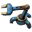Posts: 171
Joined: Wed Jan 18, 2017 2:33 am
Contact:

### Re: The most complicated Equation you can think of

keyboardhack wrote:I cheated. My computer came up with this one. You may get an error if you try run the function depending on the precision of the program running it.

f(x) = 1+(cos(ln(x/exp((x+x^arccos(sqrt(1/(arcsin(ln(tan(sqrt(ln(exp(2*x/(Pi-2*arccos(cos(arcsin(x)^2/(1+arcsin(x)^2)))))-x)/ln(10)))+1)/ln(10))+tan(sin(x^(1/sqrt(1+ln((1/2)*Pi-1)^2/ln(10)^2)-1)))-tan(ln(ln(-arctan(x*(arcsin(x*tan(exp(exp(1))))-1))))*sqrt(exp(x/sqrt(sin(exp(1)*x)))+arctan(arctan((x^arctan((1/2)*ln(arccos(arcsin(ln(arctan(arctan(Pi/x)))))^x)/ln(10))+(1/2)*ln(arccos(arccos(sqrt(sin(2*ln(exp(exp(tan((x/sqrt(-x^2+1))^x))))/ln(10))))))/ln(10))/tan(tan(exp(2^tan(sin(exp(arccos(cos(ln(cos(cos(exp(exp(x)))))))^x)+1)))))^(x/arcsin(tan(exp(1))))))))))))/x))/ln(10))+ln(exp(exp(1/sqrt(1+4*sin(sqrt(cos(cos(-1+x))))^2)))+1))^arccos(x)

f(1) = 2
which represents?

Laplace transforms which are used in aerospace mechanics technically extend to infinity depending on how much accuracy you want. The only thing possibly longer would be a power set of Laplace transforms which would have no practical use.

DerivePi
Filter InserterPosts: 504
Joined: Thu May 29, 2014 4:51 pm
Contact:

### Re: The most complicated Equation you can think of

Maybe not the most complicated equation I've experienced (diff EQ is all a blur now), but for solving local max/mins of cubic functions, I got Excel to solve for the real solution(s) to quartic functions in real time.

MrNardKing
Burner InserterPosts: 12
Joined: Sun Mar 12, 2017 8:05 pm
Contact:

### Re: The most complicated Equation you can think of

1+1=2
My computer couldn't even handle this one!
So I had to do it by hand.. took me hours.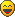Roses are red
Violets are blue
Pollution smells great

The Eriksonn
Fast InserterPosts: 229
Joined: Wed Jun 08, 2016 6:16 pm
Contact:

### Re: The most complicated Equation you can think of

Me solving derivativs in the most complicated way:

d/dx x/(1-x^-4) =

lim h->0
((hx^4-(x+h))(x^4+4h(x^3)+6(h^2)(x^2)+4xh^3+h^4)+x^5)/
((h(x^4)-h)(x^4+4h(x^3)+6(h^2)(x^2)+4xh^3+h^4)-(x^4)((h(x^4)-h)/x^4))

=(x^8-5x^4)/(x^8-2x^4+1)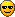mrvn
Smart Inserter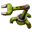Posts: 3386
Joined: Mon Sep 05, 2016 9:10 am
Contact:

### Re: The most complicated Equation you can think of

sorry, the message board won't let me paste such a long message

Pinnakle
Burner InserterPosts: 6
Joined: Fri Mar 24, 2017 9:04 pm
Contact:

### Re: The most complicated Equation you can think of

Mrmeguyme- wrote:Try and come up with the most complicated equation you can think of.
1+1= error

Koub
Global Moderator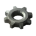Posts: 4788
Joined: Fri May 30, 2014 8:54 am
Contact:

### Re: The most complicated Equation you can think of

The equation that describes how women work.
Koub - Please consider English is not my native language.

Pinnakle
Burner InserterPosts: 6
Joined: Fri Mar 24, 2017 9:04 pm
Contact:

### Re: The most complicated Equation you can think of

Koub wrote:The equation that describes how women work.
Not even the best computers can do such a large task.

Optera
Smart InserterPosts: 2088
Joined: Sat Jun 11, 2016 6:41 am
Contact:

### Re: The most complicated Equation you can think of

Pinnakle wrote:
Koub wrote:The equation that describes how women work.
Not even the best computers can do such a large task.
Woman take time and money
Woman = Time * Money

We all know time is money
Time = Money

Therefore we can substitute
Woman = Money^2

finally since Money is the root of all evil
sqrt(Evil) = Money

substitute again and we get
Woman = Evil

mrvn
Smart InserterPosts: 3386
Joined: Mon Sep 05, 2016 9:10 am
Contact:

### Re: The most complicated Equation you can think of

radev222 wrote:The most complicated Equation is E=mc2
e^pi*i + 1 = 0

The Eriksonn
Fast InserterPosts: 229
Joined: Wed Jun 08, 2016 6:16 pm
Contact:

### Re: The most complicated Equation you can think of

e^xi=cos(x)+i*sin(x)

Aramorgot
Manual Inserter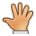Posts: 3
Joined: Wed Mar 29, 2017 8:13 pm
Contact:

### Re: The most complicated Equation you can think of

What about the legendary answer to life the universe and everything being 42?

Koub
Global ModeratorPosts: 4788
Joined: Fri May 30, 2014 8:54 am
Contact:

### Re: The most complicated Equation you can think ofKoub - Please consider English is not my native language.

noliVe
Filter InserterPosts: 325
Joined: Tue May 24, 2016 7:46 am
Contact:

### Re: The most complicated Equation you can think of

@koub where you found my classnotes from kindergarden?

### Who is online

Users browsing this forum: No registered users